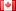# Revision history [back]

### How to construc a generator point of an elliptic curve?

I have taht elliptic curve defined in sage:

E1=EllipticCurve(GF(p),[1,0,0,333,6166])

How can i construct a generator point of an elliptic curve?

Thank you very much.

### How to construc a generator point of an elliptic curve?

I have taht elliptic curve defined in sage:

E1=EllipticCurve(GF(p),[1,0,0,333,6166])E1=EllipticCurve(GF(8209),[1,0,0,333,6166])

How can i construct a generator point of an elliptic curve?

Thank you very much.3 retaggedDSM5242 ●21 ●68 ●112

### How to construc a generator point of an elliptic curve?

I have taht elliptic curve defined in sage:

E1=EllipticCurve(GF(8209),[1,0,0,333,6166])

How can i construct a generator point of an elliptic curve?

Thank you very much.